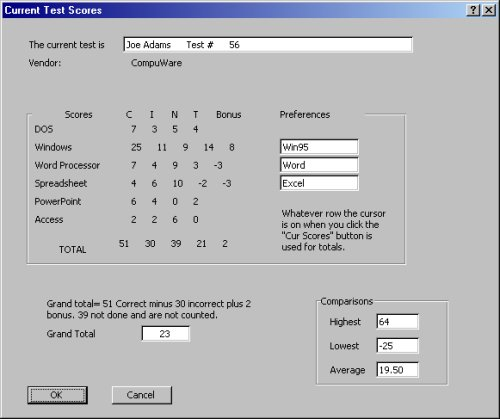# Excel VBA

 Home Excel Power BI Access SQL Word JavaScript Links Alan FAQ css navigation by Css3Menu.com

## Populate Dialog

We have this test for new people in my office. While I do not have control of what is on the test, I wrote and re-wrote (ad infinium) the spreadsheet for scoring. Basically, a candidate takes the test and the office assistant who is usually not technical and does not know or care about the answers; transfers the choices to the spreadsheet. In just a few minutes, the scores are tallied and a hiring decision can be made.

I got stuck with this because I made the highest score they had seen and I pointed out errors in their test. In my interview, they said, “Okay, you’re hired! After training you will fix the test.” Then I was stuck with it for 3 years because no one wanted to learn VBA.

This example shows the scoring dialog that the assistant is presented after transferring all the answers. While preparing this example, I saw a few things I plan to repair the next time I can go into the code. This was written for Excel 7.0/95 and works well in Excel 2000.

```Option Explicit

Sub GetScore()
Dim Dlog, NameLabel, LastRow, Vendor, DOS, Winders, WordProc
Dim Spread, PPT, Access, Scen, Totl, Grand
Dim WinPref, WPpref, SpreadPref, Spacer, TotlC, TotlI, TotlN, TotlB, TotlT, Nuts
Dim HighScore, LowScore, AveScore
Spacer = Chr(32) & Chr(32) & Chr(32) & Chr(32) & Chr(32) & Chr(32)
Sheets("Results").Activate
Nuts = Application.CountA(ActiveSheet.Range("A:A"))
LastRow = ActiveCell.Row
If LastRow > Nuts Then      'Cursor is not on a used row
MsgBox "You must have the cursor on a valid row"
Exit Sub
End If
NameLabel = Cells(LastRow, 1).Value
Vendor = Cells(LastRow, 3).Value
DOS = Cells(LastRow, 11).Value & Spacer & Cells(LastRow, 12).Value & _
Spacer & Cells(LastRow, 13).Value & Spacer & Cells(LastRow, 14).Value
Winders = Cells(LastRow, 16).Value & Spacer & Cells(LastRow, 17).Value & _
Spacer & Cells(LastRow, 18).Value & Spacer & Cells(LastRow, 19).Value _
& Spacer & Cells(LastRow, 20).Value
WinPref = Cells(LastRow, 21).Value
WPpref = Cells(LastRow, 28).Value
WordProc = Cells(LastRow, 23).Value & Spacer & _
Cells(LastRow, 24).Value & Spacer & Cells(LastRow, 25).Value _
& Spacer & Cells(LastRow, 26).Value & Spacer & Cells(LastRow, 27).Value
Spread = Cells(LastRow, 30).Value & Spacer & Cells(LastRow, 31).Value _
& Spacer & Cells(LastRow, 32).Value & Spacer & _
Cells(LastRow, 33).Value & Spacer & Cells(LastRow, 34).Value
PPT = Cells(LastRow, 37).Value & Spacer & Cells(LastRow, 38).Value _
& Spacer & Cells(LastRow, 39).Value & Spacer & Cells(LastRow, 40).Value
Access = Cells(LastRow, 42).Value & Spacer & Cells(LastRow, 43).Value _
& Spacer & Cells(LastRow, 44).Value & Spacer & Cells(LastRow, 45).Value
Scen = Spacer
SpreadPref = Cells(LastRow, 35).Value
HighScore = Application.Max(Range(Cells(3, 7), Cells(LastRow, 7)))
LowScore = Application.Min(Range(Cells(3, 7), Cells(LastRow, 7)))
AveScore = Application.Text(Application.Average(HighScore, LowScore), "#0.00;-#0.00")
TotlC = Application.Sum(Cells(LastRow, 11).Value, Cells(LastRow, 16) _
.Value, Cells(LastRow, 23), Cells(LastRow, 30), Cells(LastRow, 37), _
Cells(LastRow, 42))
TotlI = Application.Sum(Cells(LastRow, 12).Value, Cells(LastRow, 17) _
.Value, Cells(LastRow, 24), Cells(LastRow, 31), Cells(LastRow, 38), _
Cells(LastRow, 43))
TotlN = Application.Sum(Cells(LastRow, 13).Value, Cells(LastRow, 18) _
.Value, Cells(LastRow, 25), Cells(LastRow, 32), Cells(LastRow, 39), _
Cells(LastRow, 44))
TotlT = Application.Sum(Cells(LastRow, 14).Value, Cells(LastRow, 19) _
.Value, Cells(LastRow, 26), Cells(LastRow, 33), Cells(LastRow, 40), _
Cells(LastRow, 45))
TotlB = Application.Sum(Cells(LastRow, 20).Value, Cells(LastRow, 27) _
.Value, Cells(LastRow, 34))
Grand = Cells(LastRow, 7).Value
Set Dlog = DialogSheets("dlgScores")
With Dlog	'Populate the dialog
.EditBoxes("Edit Box 39").Text = NameLabel & Spacer & _
"Test #" & Spacer & Cells(LastRow, 6).Value
.Labels("Label 7").Text = Vendor
.Labels("Label 16").Text = DOS
.Labels("Label 18").Text = Winders
.Labels("Label 19").Text = WordProc
.Labels("Label 20").Text = Spread
.Labels("Label 21").Text = PPT
.Labels("Label 22").Text = Access
.Labels("Label 14").Text = "Grand total= " & TotlC & _
" Correct minus " & TotlI & " incorrect plus " & _
TotlB & " bonus. " & TotlN & " not done and are not counted."
.Labels("Label 24").Text = TotlC & Spacer & TotlI & Spacer _
& TotlN & Spacer & TotlT & Spacer & TotlB
.EditBoxes("Edit Box 31").Text = WinPref
.EditBoxes("Edit Box 30").Text = WPpref
.EditBoxes("Edit Box 33").Text = Spacer & Grand   'Center in box
.EditBoxes("Edit Box 32").Text = SpreadPref
.EditBoxes("Edit Box 44").Text = HighScore        '06/13/2000
.EditBoxes("Edit Box 45").Text = LowScore
.EditBoxes("Edit Box 46").Text = AveScore
End With
Application.ScreenUpdating = False
Dlog.Show
End Sub```